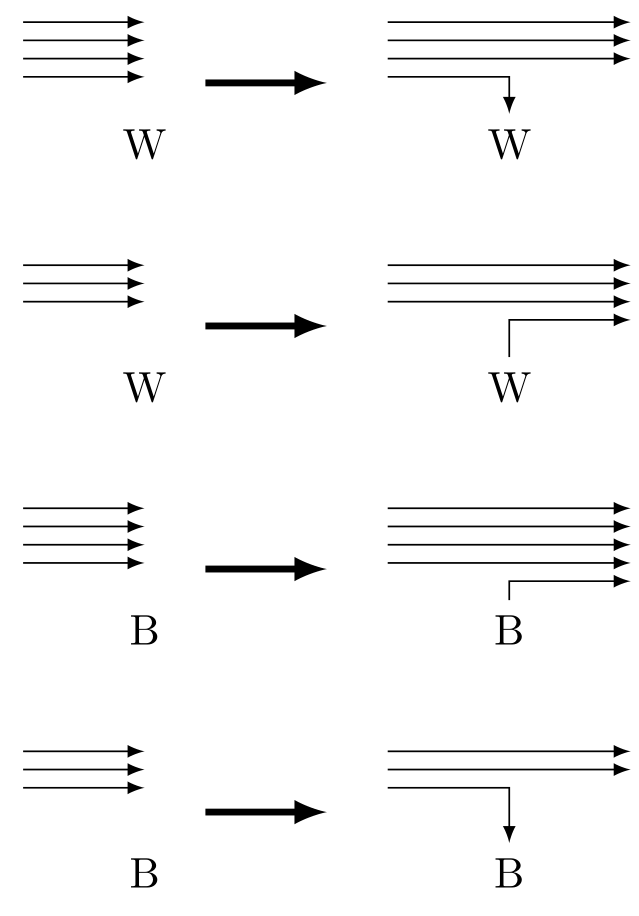# Japanese Student Championship 2019 Qualification C - Cell Inversion

Looking at elements from left to right, we'll decide to open or close an interval. We can determine which operation we should do by the parity of the number of intervals currently open and the current character. We can choose the movement of neither open nor close because if we do such a operation, the number of operations cannot be N.\documentclass[dvipdfmx,margin=2mm]{standalone}
\usepackage{tikz}
\begin{document}

\begin{tikzpicture}[foo/.style={circle,draw=transparent,fill=black,inner sep=0,minimum size=1mm}]
\begin{scope}
\begin{scope}
\node(0) at (0,0) {W};
\draw[-latex] (-1,1) -- (0,1);
\draw[-latex] (-1,0.85) -- (0,0.85);
\draw[-latex] (-1,0.7) -- (0,0.7);
\draw[-latex] (-1,0.55) -- (0,0.55);
\end{scope}
\begin{scope}[xshift=3cm]
\node(0) at (0,0) {W};
\draw[-latex] (-1,1) -- (1,1);
\draw[-latex] (-1,0.85) -- (1,0.85);
\draw[-latex] (-1,0.7) -- (1,0.7);
\draw[-latex] (-1,0.55) -- (0,0.55) -- (0);
\end{scope}
\draw[ultra thick,-latex] (0.5,0.5)--(1.5,0.5);
\end{scope}

\begin{scope}[yshift=-2cm]
\begin{scope}
\node(0) at (0,0) {W};
\draw[-latex] (-1,1) -- (0,1);
\draw[-latex] (-1,0.85) -- (0,0.85);
\draw[-latex] (-1,0.7) -- (0,0.7);
\end{scope}
\begin{scope}[xshift=3cm]
\node(0) at (0,0) {W};
\draw[-latex] (-1,1) -- (1,1);
\draw[-latex] (-1,0.85) -- (1,0.85);
\draw[-latex] (-1,0.7) -- (1,0.7);
\draw[-latex] (0) -- (0,0.55) -- (1,0.55);
\end{scope}
\draw[ultra thick,-latex] (0.5,0.5)--(1.5,0.5);
\end{scope}

\begin{scope}[yshift=-4cm]
\begin{scope}
\node(0) at (0,0) {B};
\draw[-latex] (-1,1) -- (0,1);
\draw[-latex] (-1,0.85) -- (0,0.85);
\draw[-latex] (-1,0.7) -- (0,0.7);
\draw[-latex] (-1,0.55) -- (0,0.55);
\end{scope}
\begin{scope}[xshift=3cm]
\node(0) at (0,0) {B};
\draw[-latex] (-1,1) -- (1,1);
\draw[-latex] (-1,0.85) -- (1,0.85);
\draw[-latex] (-1,0.7) -- (1,0.7);
\draw[-latex] (-1,0.55) -- (1,0.55);
\draw[-latex] (0) -- (0,0.4) -- (1,0.4);
\end{scope}
\draw[ultra thick,-latex] (0.5,0.5)--(1.5,0.5);
\end{scope}

\begin{scope}[yshift=-6cm]
\begin{scope}
\node(0) at (0,0) {B};
\draw[-latex] (-1,1) -- (0,1);
\draw[-latex] (-1,0.85) -- (0,0.85);
\draw[-latex] (-1,0.7) -- (0,0.7);
\end{scope}
\begin{scope}[xshift=3cm]
\node(0) at (0,0) {B};
\draw[-latex] (-1,1) -- (1,1);
\draw[-latex] (-1,0.85) -- (1,0.85);
\draw[-latex] (-1,0.7) -- (0,0.7) -- (0);
\end{scope}
\draw[ultra thick,-latex] (0.5,0.5)--(1.5,0.5);
\end{scope}
\end{tikzpicture}

\end{document}